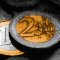Savings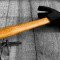DIY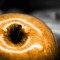Health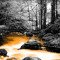EcologyScience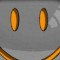Fun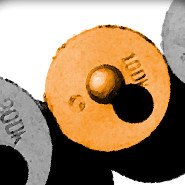# Convert weights or masses

Convert weights or masses with the most commonly used units. Possible conversions in metric system, imperial / U.S. customary system and apothecaries' system.

t
kN
kg
N
ct
g
mg
mcg
u

## Info

In most modern scientific work, physical quantities are measured in SI units. The SI unit of weight is the same as that of force: the newton (N) – a derived unit which can also be expressed in SI base units as kg⋅m/s2 (kilograms times meters per second squared).

In commercial and everyday use, the term "weight" is usually used to mean mass, and the verb "to weigh" means "to determine the mass of" or "to have a mass of". Used in this sense, the proper SI unit is the kilogram (kg). Pound and other non-SI units In United States customary units, the pound can be either a unit of force or a unit of mass. Related units used in some distinct, separate subsystems of units include the poundal and the slug. The poundal is defined as the force necessary to accelerate an object of one-pound mass at 1 ft/s2, and is equivalent to about 1/32.2 of a pound-force. The slug is defined as the amount of mass that accelerates at 1 ft/s2 when one pound-force is exerted on it, and is equivalent to about 32.2 pounds (mass). Source : Wikipédia.org